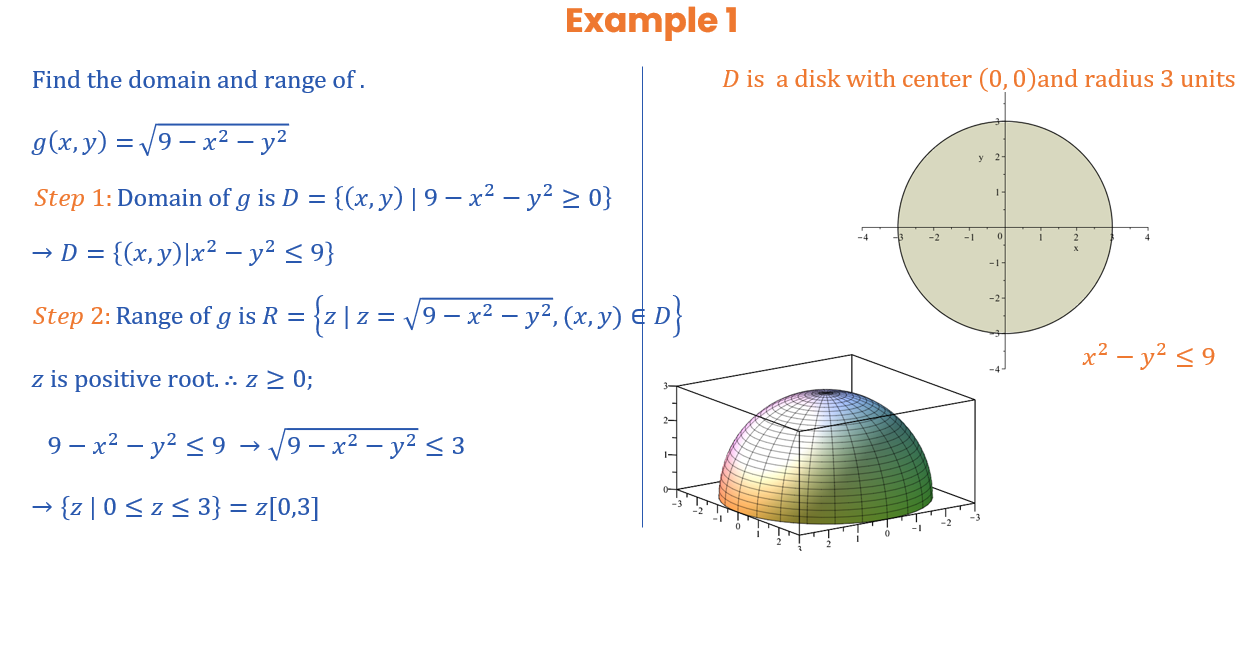## Ramakrishnan

Ramakrishnan Vaidyanathan

## 394 Reputation

8 years, 204 days

## Social Networks and Content at Maplesoft.com

With twenty years of Industrial experience and twenty years of teaching experience, I am now as retired Professor, using Maple to teach mathematics subject for students studying X to XII standards. Published XII Mathematics books.

## quo and rem - usage explained...

Thank you Dharr,

It will be useful when explaining to students, division and remainder..

RK

## Graphical visualizations for any solutio...

For integral problems if graphical plots are included by default with default parameter values where possible would be great help to Design of Experiments experts and for those wanting to use RSM (Response Surface Methods) for optimizing parameters for product quality. One need to learn a deep extent to do these things in maplesoft. It is just an opinion. The product is anyway an excellent tool and answers complicated problems as of now. Cheers. Ramakrishnan

## solving polynomials...

Is not this simple solve command give the solutions?

I have attached the doc for help.(1)(2)## TextFont...

Dear sir,

Text font can be anything as you like.

I have used Vivaldi style font here.

Hope this is useful.

Ramakrishnan,
plots:-textplot({[4.2, 1.9, sin(nprintf(#mn("%a&deg;");, 30))]}, symbol = point, color = black, font = ["Vivaldi", "Bold", 25]);

Dear sir,

In my opinion, (3,4) and (4, 3) are pairs of numbers giving the domain points by coordinates (x, y).

We say (x, y) < (3, 4) which means x takes values less than 3 and y takes values less than 4

OR or (x, y) < (4, 3)  which means x takes values less than 4 and y takes values less than 3

For all the points in the domain, valid function can be prescribed. the function gives valid unique numbers for each pair of the points in the given domain.

Comparing two coordinate values can be done differently as mentioned , though not done generally.

Comparing coordinates (3, 4) < (4, 3) results in false as 4 is >3.

Comparing coordinates (3, 4) < (5, 7) results in true as 3 < 5 and 4 < 7.

Thanks.

Ramakrishnan V

## More on two dimensional domain...

Dear sir,

The domain data and function described by me are just a sample and not to do with any expected results.

In fact I was interested in feeding the doamain ( x and y range and the valid  function for this domain) to get the expected image from the maple.

In fact carl love has given a solution to get a plot of hemisphere, which is the answer for my earlier question.

I wanted to know  how to give two dimensional piece wise function in maple for the same and plot.

I attach relevent documents just for reference.

plot of a hemisphere with input domain (x,y) as a circle of radius 3 cm and function representing hemisphere (sphere) as mathematical relation x sq + y sq = r sq

plot3d(-sqrt(-x^2 - y^2 + 9), y = -sqrt(-x^2 + 9) .. sqrt(-x^2 + 9), x = -3 .. 3, scaling = constrained)

recommended by Dr. Robert Lopez is a very good way of describing the domain and function and it displayed perfect hemisphere (upside as well as down side).

The plot command recommended by you is very interesting, but an explanation for the plot3d statement would very much help me. Like the " 'and ' and ' + ' notation purpose. What does u stand for ? Are all u, v, a and b refer to intervals?

Thanks for the immense help.

Ramakrishnan V

plot3d(-sqrt(x^2 - y^2 + 9), y = -sqrt(-x^2 + 9) .. sqrt(-x^2 + 9), x = -3 .. 3, scaling = constrained);